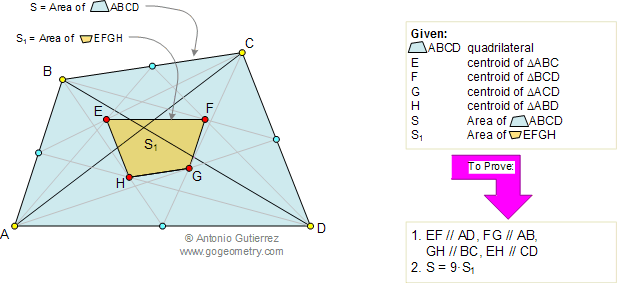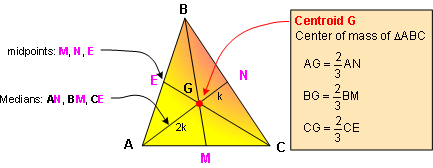# Geometry Problem 98: Exploring Quadrilateral Properties: Areas, Centroids, and Similarity

 Consider the diagram below, which displays a quadrilateral ABCD of area S. The triangles ABC, BCD, ACD, and ABD have centroids E, F, G, and H respectively. Let S1 be the area of the quadrilateral EFGH. It can be proven that (1) EF, FG, GH, and EH are parallel to AD, AB, BC, and CD respectively, and (2) S = 9S1. View or post a solution. .Geometric shapesCentroids, areas, similarityA world of wonders

FACTS AND HINTS:

1. CENTROID:
The geometric centroid (center of mass) or barycenter of the polygon vertices of a triangle is the point G which is also the intersection of the triangle's three triangle medians.

Proposition: The centroid divides each of the medians in the ratio 2:12. PROPORTIONAL SEGMENTS:

Proposition 1: If a line is parallel to one side of a triangle, then it divides the other two sides proportionally.

Proposition 2: If a line divides two sides of a triangle proportionally, it is parallel to the third side. (Converse of -proposition 1.)

3. SIMILAR POLYGONS - Ratio of Areas:

Proposition: If two polygons are similar, the ratio of their areas is equal to the square of the ratio of their corresponding sides.

Home | Geometry | Search | Problems | Similarity | Wolfram Demonstrations Project | Parallelograms | All Problems | 91-100 | Visual Index | Email | View or post a solution | by Antonio Gutierrez